### Home > MC2 > Chapter 5 > Lesson 5.3.2 > Problem5-103

5-103.
1. Look at the algebra tile shape below. Homework Help ✎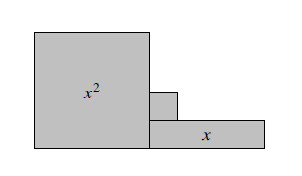1. Write an algebraic expression for the perimeter of the shape in two ways, first by finding the length of each of the sides and adding them all together and then by writing an equivalent, simplified expression.

2. Write an algebraic expression for the area of the shape.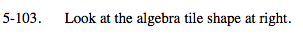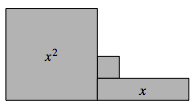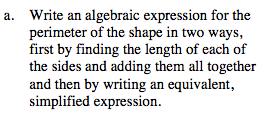Label all the side lengths.

x + x + x − 2 + 1 + 1 + x − 1 + 1 + x + x

Simplify this expression.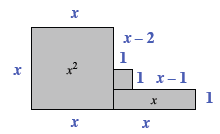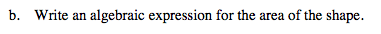To find the area, add up the values of all the tiles within the perimeter.